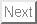Academic Paper

Abstract: This paper proposes a calculation method of local bifurcation points for discrete-time dynamical systems with piecewise nonlinear characteristic (PNDDS). First, an n-dimensional PNDDS, which has two piecewise nonlinear maps, is shown and its variational equation is defined. Next, the calculation method of the local bifurcation points is proposed using the conditional equation of the periodic solution and the characteristic equation. Here, we have to calculate derivatives of the map with an initial value and with a bifurcation parameter to obtain the bifurcation points continuously in the parameter space. The above calculation process is a key of the proposed method, and we explain it in detail. Finally, we apply the proposed method to a two-dimensional PNDDS and calculate the local bifurcation points for confirming its validity.

Contact address: ueta @ tokushima-u.ac.jp
2020-10-01